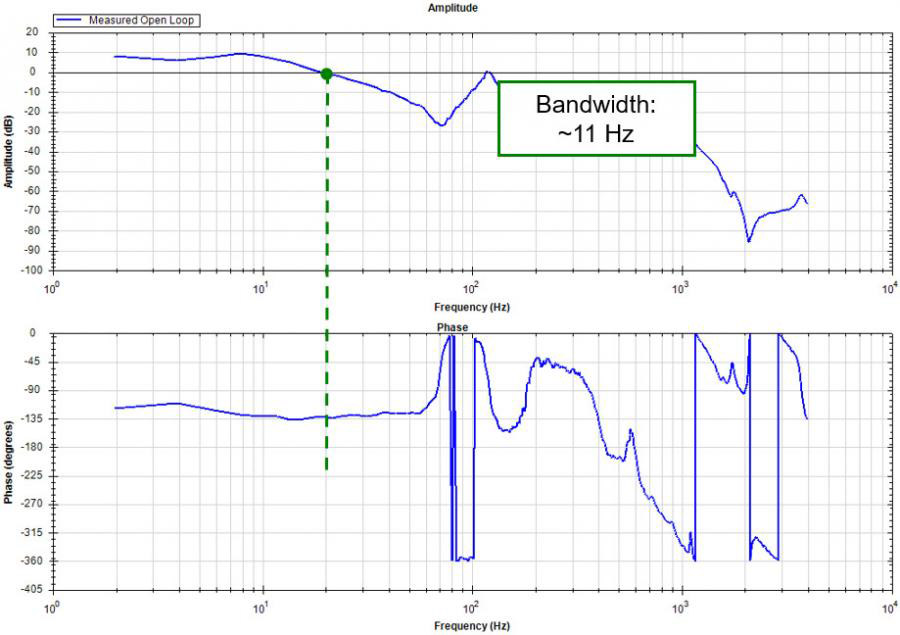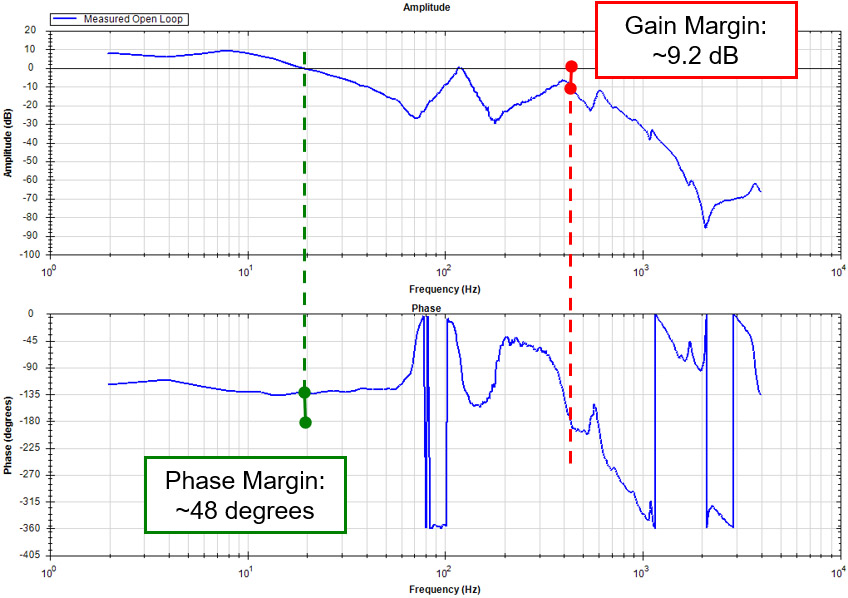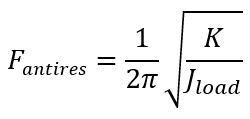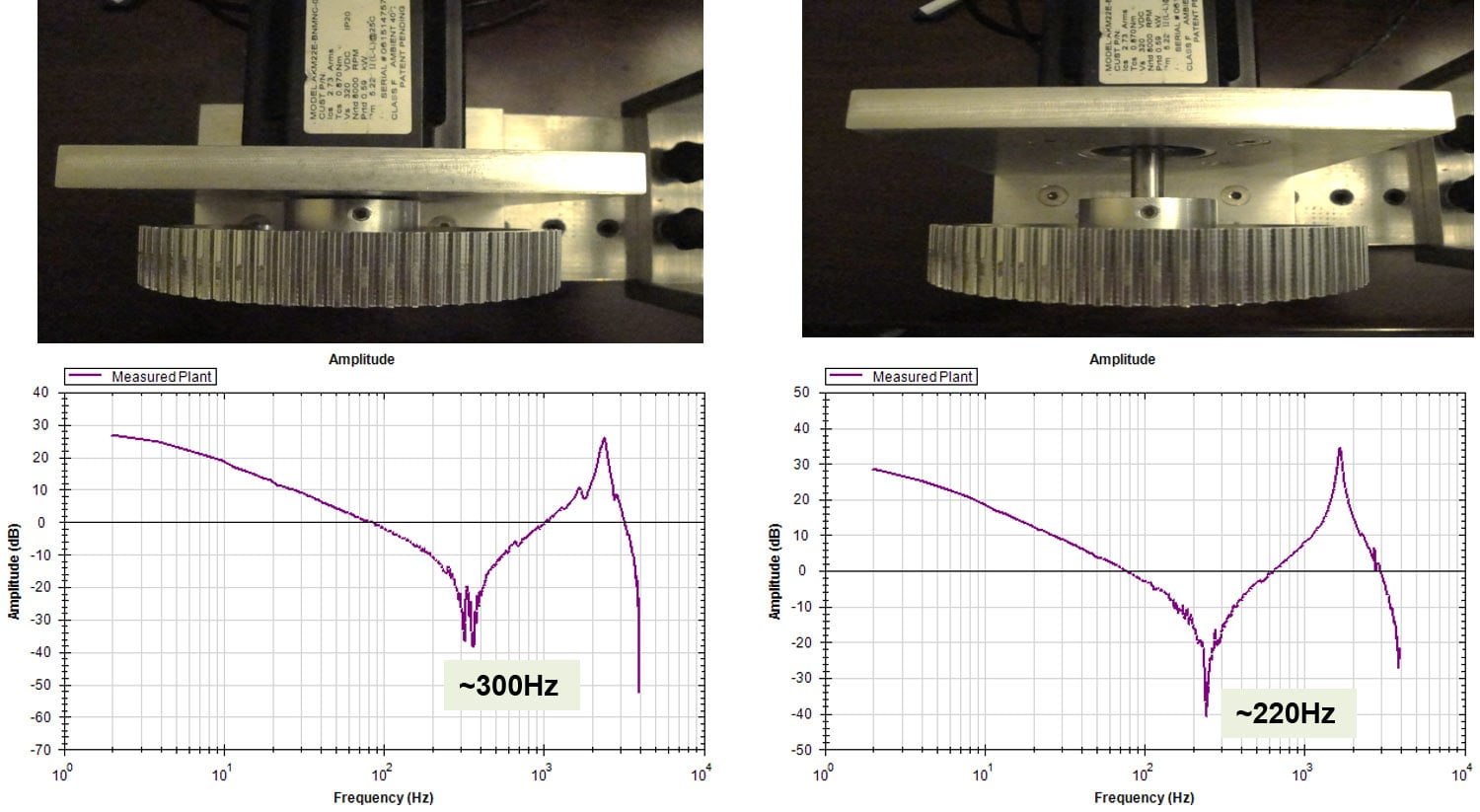# Four Key Values to Determine Servo System Stability

13 Nov 2020
Kollmorgen Experts

A stable servo system is essential for optimal machine performance, durability, safety, and consistent machine performance, which improves overall machine operation. Servo systems operate in various degrees of stability, with a fine line between stable and unstable. Changes in the mechanical system over time or machines with varying loads can move the system from a stable to unstable operating condition. This article unveils four key values that determine the stability level of a servo system.

The Bode plot, a key analytical tool to determine the stability level of a servo system, provides a visual depiction of the mechanism in the frequency domain and identifies the phase and amplitude response of a series of injected signals into the system. From this plot, four values - bandwidth, amplitude gain margin, phase margin, and stiffness - can be calculated to provide a reasonable determination of servo stability. The phase and gain margins determine specifically how close the mechanism is to becoming unstable.

Bandwidth of a servo system identifies the frequency at which the servo system can follow a given command and maintain a specified level of performance. It is an indication of how quickly the system can respond to a motion command and then stabilize (settle). In the frequency domain, the bode plot provides a direct measurement of the closed loop bandwidth where the open loop amplitude gain plot crosses 0 dB. (See figure 1)Figure 1 - Bandwidth Measurement

The amplitude plot is a measurement of the reduction in the response amplitude as the input frequency is increased. The amplitude gain margin is the value below 0 dB where the phase plot first crosses -180 degrees. The further the value is below 0 dB, the more stable the system. In figure 2 shown below, the amplitude gain for the system is -9.8 dB.

The phase plot is a measurement of the phase response in degrees as the input frequency is increased. At a certain frequency, the response to the input command will be 180 degrees out of phase. To determine the phase margin, calculate the difference between -180 degrees and the phase value at which the amplitude plot crosses 0 dB. The larger this value, the more stable the system will be. In the chart below, the phase margin is 48 degrees. The typical values that represent a stable servo system are an amplitude gain margin of ~ -8 dB and a phase margin of 45 degrees.Figure 2 - Phase and Gain Margins

The final measurement of a stable system is the stiffness of the mechanical system. Stiffness is important in obtaining the highest possible bandwidth - the stiffer the system, the higher the bandwidth that can be achieved. The bode plot provides a quick snapshot of the system stiffness. The lower the frequency of the first anti-resonant node, the less stiff the system. The first anti-resonant frequency is represented by the following equation:Given a fixed load (Jload), as the stiffness (K) decreases, the first anti-resonant frequency decreases. This in turn reduces the possible bandwidth. Reduced bandwidth may affect optimal performance of a machine, or at best, limit productivity.

The image below shows how even a change in position of a pulley on a shaft can affect the mechanical stiffness and raise or lower the first anti-resonant frequency.These four key elements of servo stability provide a blueprint for machine performance optimization. The phase and gain margin measurements provided by the bode plots, along with the bandwidth, stiffness, and overall mechanism resonances, help the servo technician use various filtering techniques to tune the system. A well-tuned and stable servo system is key to machine performance, durability, and safety.

### More on This Topic

What is Bandwidth?

What is Amplitude Gain Margin?

What is Phase Margin?This blog was a collaborative effort among a team of motion and automation experts here at Kollmorgen, including engineers, customer service and design experts. Wherever you are in your project, we’re here to help.

Aerospace & Defense
Applications
Automated Guided Vehicles
Embedded Motion
Engineering
Fieldbus
Food Regulations
General
History
Installation Tips
Interconnectivity
Medical
Oil and Gas
Packaging
Robotics
Technology
University Partnerships